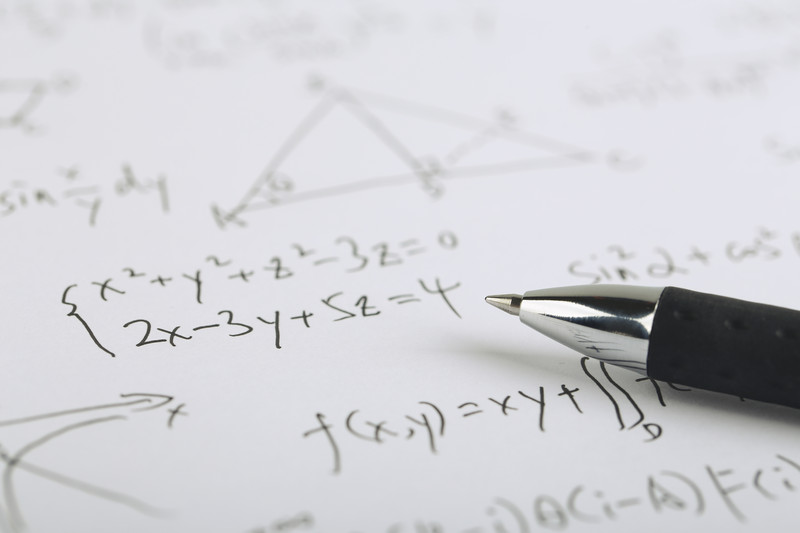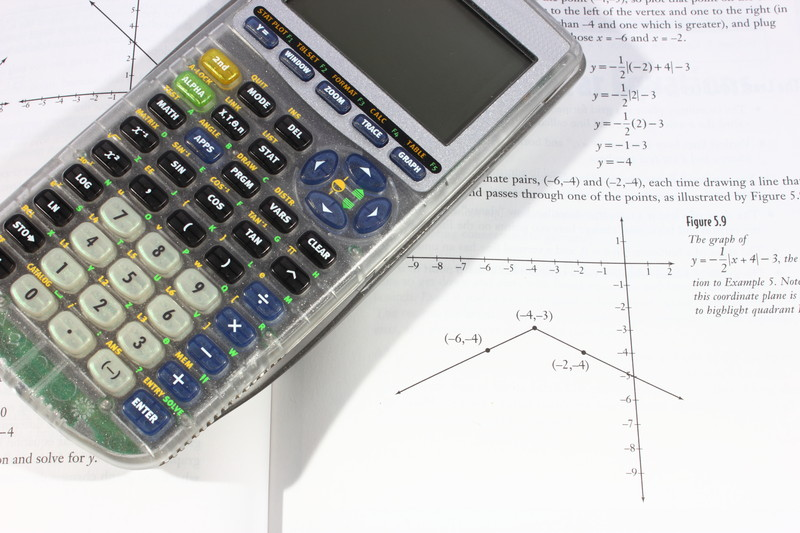## Lesson 1: Solving PSAT Equations and Word ProblemsTackle foundational algebra topics that will yield big points on test day.

• #### Math Strategies and Linear Equations

Applying the same basic steps to every PSAT math question—whether it asks you about geometry, algebra, or even trigonometry—will help you avoid minor mistakes as well as tempting wrong answer choices.

• #### Linear Equations Practice Questions

Linear equations and linear graphs are some of the most common test questions on the PSAT Math Test. Linear Equations can be used to model relationships and changes such as those concerning time, temperature, or population.

• #### Linear Word Problems

On the PSAT Math Test, another way linear equations can be made to look complicated is for them to be disguised in “real-world” word problems, where it’s up to you to extract and solve an equation.

• #### Linear Graphs

On the PSAT Math Test, working with equations algebraically is only half the battle. The PSAT Math Test will also expect you to work with graphs of linear equations, which means using lines in slope-intercept form and point-slope form.

• #### Quiz: Equations and Word Problems

Take a free 10-minute, 5 question quiz to test your skills from lesson 1!

## Lesson 2: PSAT Problem Solving: Rates, Ratios and MoreLearn Kaplan methods to conquer questions about PSAT problem solving questions, including rates, ratios, proportions, and percentages.

• #### Systems of Equations

Learn to distinguish between independent and dependent equations and solve two-variable systems of linear equations.

• #### Combination and Substitution

Let’s look at some methods for solving these systems effectively. The two main methods for solving a system of linear equations are substitution and combination.

• #### Word Problems and Multiple Equations

While solving systems of equations can be relatively straightforward once you get the hang of it, sometimes you’ll encounter a complex word problem and need to translate it into a system of equations and then solve.

• #### Quiz: Systems of Equations

Take a free 10-minute, 5 question quiz to test your skills on Systems of Equations.

• #### Rates and Measurements

The PSAT Math Test contains multiple-choice and grid-in questions, as well as multi-part math question sets. These question sets have multiple parts that are based on the same scenario and may require more analysis and planning than a typical multiple-choice question

• #### Ratios and Proportions

Ratios and proportions are quite common in everyday life. Whether it’s making a double batch of meatballs or calculating the odds of winning the lottery, you’ll find that ratios and proportions are invaluable in myriad situations.

• #### Percentages

Percentages aren’t just for test grades; you’ll find them frequently throughout life—discount pricing in stores, income tax brackets, and stock price trackers all use percents in some form. It’s critical that you know how to use them correctly, especially on Test Day.

• #### Quiz: Rates, Ratios, Proportions and Percents

Take a free 10-minute, 5 question quiz to test your skills on these highly tested math concepts.

## Lesson 3: PSAT Math Rules: Exponents, Functions and MoreBreak down the critical algebra and function rules that will help you confidently tackle exponents, radicals, and more

We often turn to our calculators to solve difficult radical and exponent problems, especially in math-intensive classes. However, being too calculator dependent can cost you time and points on the PSAT. Further, on the PSAT, many radical and exponent problems are structured in such a way that your calculator can’t help you, even if it is allowed.

• #### Polynomials

An important topic you are sure to see on the PSAT is polynomials. A polynomial is an expression comprised of variables, exponents, and coefficients, and the only operations involved are addition, subtraction, multiplication, division (by constants only), and non-negative integer exponents.

• #### Rational Expressions and Equations

A rational expression is simply a ratio (or fraction) of polynomials. In other words, it is a fraction with a polynomial as the numerator and another polynomial as the denominator. The rules that govern fractions and polynomials also govern rational expressions, so if you know these well, you’ll be in good shape when you encounter one on Test Day.

• #### Quiz: Exponents, Roots, and Polynomials

Take a free 10-minute, 5 question quiz to test your skills on exponents, roots and polynomials.

• #### Functions on the PSAT

Functions act as rules that transform inputs into outputs, and they differ from equations in that each input must have only one corresponding output.

The ability to interpret the graph of a function will serve you well on Test Day. To interpret graphs of functions, you’ll need to utilize the same skills you use to interpret “regular” equations on the coordinate plane, so this material shouldn’t be completely foreign.

• #### Function Behavior and Transformations

When describing the graph of a function or an interval (a specific segment) of a function, the trend of the relationship between the x- and y-values while reading the graph from left to right is often important. Three terms you are sure to see in more difficult function questions are increasing, decreasing, and constant. Let’s look at what these terms mean and how they apply to PSAT questions.

• #### Quiz: Functions on the PSAT

Take a free 10-minute, 4 question quiz to test your skills on PSAT functions.

### PSAT Study Plan

It’s important to come up with a game plan for how you’re going to prepare for and take the PSAT. It might seem overwhelming, but don’t worry: Kaplan’s PSAT study plan includes test info, testing tips and strategies, and study resources.